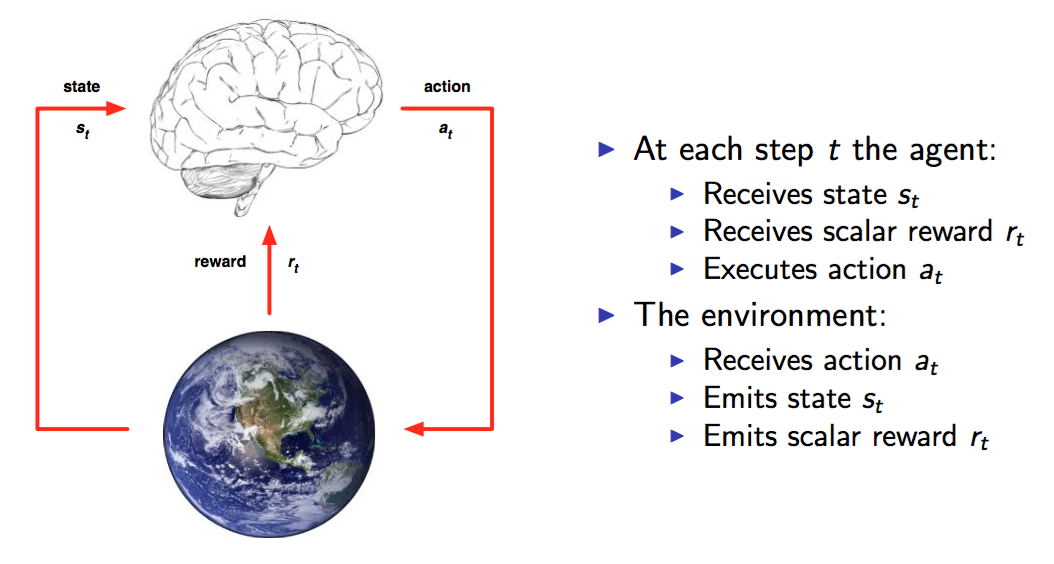# Deep Reinforcement Learning 基础知识（DQN方面）

## Introduction

Deep Reinforcement Learning因为具备真正实现AI的潜力，受到了Google等企业的关注。DeepMind 50多人的团队被Google以4亿美元的价格收购。而15年12月份刚刚由Elon Musk牵头成立的OpenAI，则一开始就获得了10亿美元的投资，而OpenAI中的好几位成员都来自UC Berkerley的Pieter Abbeel团队。

Pieter Abbeel团队紧随DeepMind之后，采用另一种方法直接实现了机器人的End-to-End学习，其成果也引起了大量的媒体报道和广泛关注。今年的NIPS 2015 更是由Pieter Abbeel及DeepMind的David Silver联合组织了Deep Reinforcement Learning workshop。可以说，目前在Deep Reinforcement Learning取得开拓性进展的主要集中在DeepMind和UC Berkerley团队。

• 深度学习特别是CNN卷积神经网络（由于感知Perception大多来自于视觉信息）
• 增强学习基础知识

## What is Reinforcement Learning 增强学习是什么a=π(s)

π(a|s)

{s1,a1,r1,s2,a2,r2,...,at1,st}

## MDP（Markov Decision Process）马尔科夫决策过程

The future is independent of the past given the present

P(st+1|st)=P(st+1|s1,...,st)

P为概率。

Gt=Rt+1+λRt+2+...=k=0λkRt+k+1

v(s)=E[Gt|St=s]

## Bellman Equation

v(s)=E[Gt|St=s]=E[Rt+1+λRt+2+λ2Rt+3+...|St=s]=E[Rt+1+λ(Rt+2+λRt+3+...)|St=s]=E[Rt+1+λGt+1|St=s]=E[Rt+1+λv(St+1)|St=s]

v(s)=E[Rt+1+λv(St+1)|St=s]

### Action-Value function 动作估值函数

Qπ(s,a)=E[rt+1+λrt+2+λ2rt+3+...|s,a]=Es[r+λQπ(s,a)|s,a]

### Optimal value function 最优动作估值函数

Q(s,a)=Es[r+λmaxaQ(s,a)|s,a]

### Value iteration 估值迭代

Qi+1(s,a)=Es[r+λmaxaQi(s,a)|s,a]

## Q Learning

Q Learning的思想完全根据value iteration得到。但要明确一点是value iteration每次都对所有的Q值更新一遍，也就是所有的状态和动作。但事实上在实际情况下我们没办法遍历所有的状态，还有所有的动作，我们只能得到有限的系列样本。因此，只能使用有限的样本进行操作。那么，怎么处理？Q Learning提出了一种更新Q值的办法：

Q(St,At)Q(St,At)+α(Rt+1+λmaxaQ(St+1,a)Q(St,At))

初始化 状态S
重复（对episode中的每一步）：
使用某一个policy比如（ϵgreedy$\epsilon-greedy$)根据状态S选取一个动作执行
执行完动作后，观察reward和新的状态S$S^\prime$
Q(St,At)Q(St,At)+α(Rt+1+λmaxaQ(St+1,a)Q(St,At))$Q(S_{t},A_{t}) \leftarrow Q(S_{t},A_{t})+\alpha(\color{red}{R_{t+1}+\lambda \max _aQ(S_{t+1},a)} - Q(S_t,A_t))$
SS$S \leftarrow S^\prime$
循环直到S终止

### Exploration and Exploitation 探索与利用

• 随机的生成一个动作
• 根据当前的Q值计算出一个最优的动作，这个policy π$\pi$称之为greedy policy，也就是
π(St+1)=argmaxaQ(St+1,a)

### Value function approximation 估值函数估计

1. 确定一个loss function
2. 计算w$w$关于loss function的梯度
3. 使用梯度下降比如随机梯度下降SGD等方法来更新θ$\theta$

## Deep Q-Learning

Q(s,a,w)Qπ(s,a)

### Step 2: 在Q值中使用均方差mean-square error 来定义目标函数objective function也就是loss function

L(w)=E[(r+γmaxaQ(s,a,w)TargetQ(s,a,w))2]

### Step 3：计算参数w$w$关于loss function的梯度

L(w)w=E[(r+γmaxaQ(s,a,w)Q(s,a,w))Q(s,a,w)w]